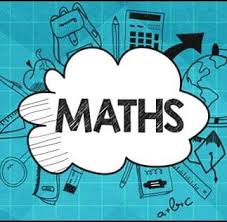# Addition

## Worksheets

SUBJECT: MATHEMATICS
LEVEL: STANDARD ONE
STRAND: NUMBERS
TOPIC: PROBLEM SOLVING WITH ADDITION

At the end of this worksheet, you will be able to:

• Solve one-step real-life addition problems, using pictorial and symbolic representation.

Key Points:

• A one-step problem requires only one mathematical operation to solve it. These include; addition, subtraction, multiplication or division problems.
• Symbolic representation of one-step addition problems involves the use of digits and include the representation “+”, which means to add.

Example: Jared had 12 marbles. His brother gave her 6 more.
How many marbles do Jared now have altogether?